Welcome to Wall Street Prep! Use code at checkout for 15% off.# Solvency Ratio

Guide to Understanding the Solvency Ratio Concept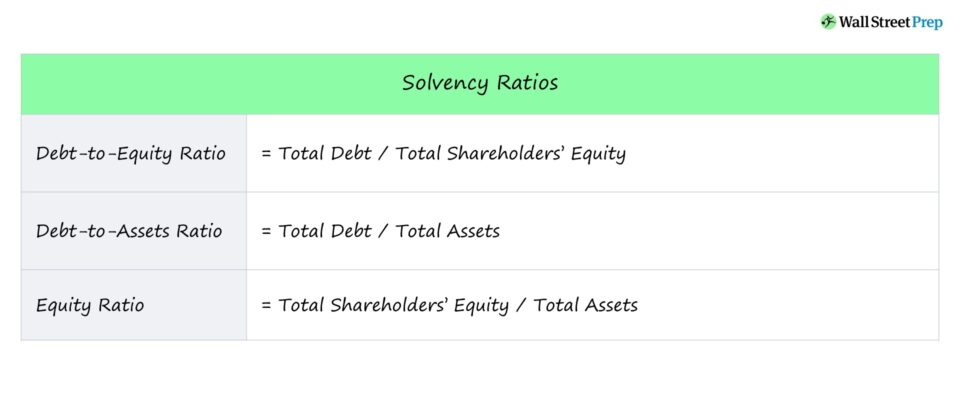## How to Calculate Solvency Ratio?

A solvency ratio assesses the long-term viability of a company – namely, if the financial performance of the company appears sustainable and if operations are likely to continue into the future.

• Liabilities: Liabilities are defined as obligations that represent cash outflows, most notably debt, which is the most frequent cause of companies becoming distressed and having to undergo bankruptcy. If debt is added to a company’s capital structure, a company’s solvency is put at increased risk, all else being equal.
• Assets: On the other hand, assets are defined as resources with economic value that can be turned into cash (e.g. accounts receivable, inventory) or generate cash (e.g. property, plant & equipment, or “PP&E”).

With that said, for a company to remain solvent, the company must have more assets than liabilities – otherwise, the burden of the liabilities will eventually prevent the company from staying afloat.

## Solvency Ratio Formula

Solvency ratios compare the overall debt load of a company to its assets or equity, which effectively shows a company’s level of reliance on debt financing to fund growth and reinvest into its own operations.

### Debt to Equity Ratio Formula (D/E)

The debt-to-equity ratio (D/E) compares a company’s total debt balance to the total shareholders’ equity account, which shows the percentage of financing contributed by creditors as compared to that of equity investors.• Higher D/E ratios mean a company relies more heavily on debt financing as opposed to equity financing – and therefore, creditors have a more substantial claim on the company’s assets if it were to be hypothetically liquidated.
• A D/E ratio of 1.0x means that investors (equity) and creditors (debt) have an equal stake in the company (i.e. the assets on its balance sheet).
• Lower D/E ratios imply the company is more financially stable with less exposure to solvency risk.

### Debt to Assets Ratio Formula (D/A)

The debt-to-assets ratio compares a company’s total debt burden to the value of its total assets.This ratio evaluates whether the company has enough assets to satisfy all its obligations, both short-term and long-term – i.e. the debt-to-assets ratio estimates how much value in assets would be remaining after all the company’s liabilities are paid off.

• Lower debt-to-assets ratios mean the company has sufficient assets to cover its debt obligations.
• A debt-to-assets ratio of 1.0x signifies the company’s assets are equal to its debt – i.e. the company must sell off all of its assets to pay off its debt liabilities.
• Higher debt-to-assets ratios are often perceived as red flags, since the company’s assets are inadequate to cover its debt obligations. This may imply that the current debt burden is too much for the company to handle.

Like the debt-to-equity ratio, a lower ratio (<1.0x) is viewed more favorably, as it indicates the company is stable in terms of its financial health.

### Equity Ratio Formula

The third solvency ratio we’ll discuss is the equity ratio, which measures the value of a company’s equity to its assets amount.The equity ratio shows the extent to which the company’s assets are financed with equity (e.g. owners’ capital, equity financing) rather than debt.

In other words, if all the liabilities are paid off, the equity ratio is the amount of remaining asset value left over for shareholders.

• Lower equity ratios are viewed as more favorable since it means that more of the company is financed with equity, which implies that the company’s earnings and contributions from equity investors are funding its operations – as opposed to debt lenders.
• Higher equity ratios signal that more assets were purchased with debt as the source of capital (i.e. implying the company carries a substantial debt load).

## What is the Difference Between Solvency Ratio vs. Liquidity Ratio?

Both solvency and liquidity ratios are measures of leverage risk; however, the major difference lies in their time horizons.

• Liquidity Ratio: Liquidity ratios are short-term oriented (i.e. current assets, short-term debt coming due in <12 months).
• Solvency Ratio: In contrast, a solvency ratio takes on more of a long-term view, i.e. the sustainability of the company and ability to continue operating as a “going concern”.

Nevertheless, both ratios are closely related and provide important insights regarding the financial health of a company.

## Solvency Ratio Calculator

We’ll now move to a modeling exercise, which you can access by filling out the form below.Submitting...

## 1. Balance Sheet Assumptions

In our modeling exercise, we’ll begin by projecting a hypothetical company’s financials across a five-year time span.

Our company has the following balance sheet data as of Year 1, which is going to be held constant throughout the entirety of the forecast.

As of Year 1, our company has \$120m in current assets and \$220m in total assets, with \$50m in total debt.

For illustrative purposes, we’ll assume the only liabilities that the company has are debt-related items, so the total equity is \$170m – in effect, the balance sheet is in balance (i.e. assets = liabilities + equity).

For the rest of the forecast – from Year 2 to Year 5 – the short-term debt balance will grow by \$5m each year, whereas the long-term debt will grow by \$10m.

## 2. Debt to Equity Ratio Calculation Analysis (D/E)

The debt-to-equity ratio (D/E) is calculated by dividing the total debt balance by the total equity balance, as shown below.

In Year 1, for instance, the D/E ratio comes out to 0.3x.

• Debt-to-Equity Ratio (D/E) = \$50m / \$170m = 0.3x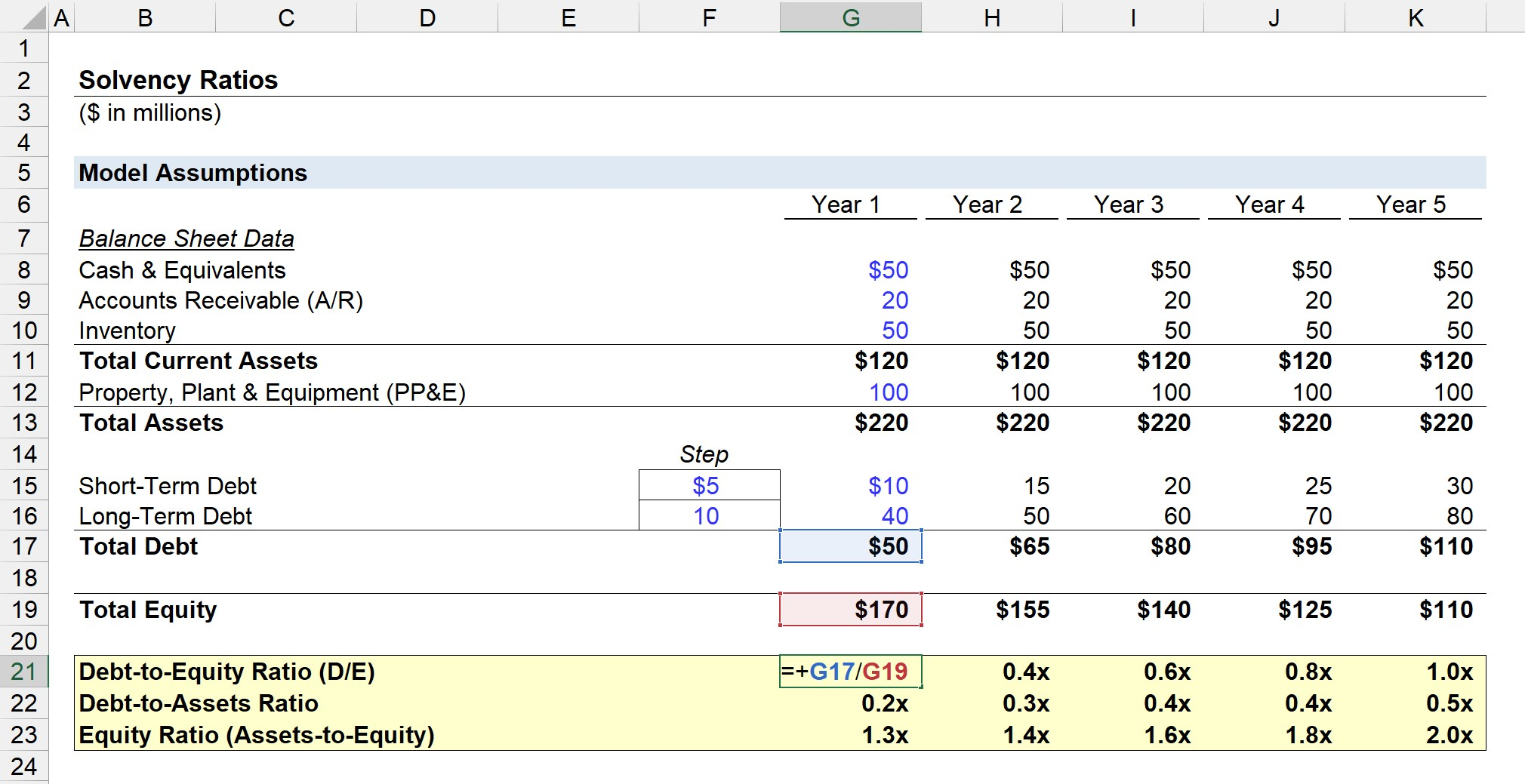## 3. Debt to Assets Ratio Calculation Analysis

Next, the debt-to-assets ratio is calculated by dividing the total debt balance by the total assets.

For example, in Year 1, the debt-to-assets ratio is 0.2x.

• Debt-to-Assets Ratio = \$50m / \$220m = 0.2x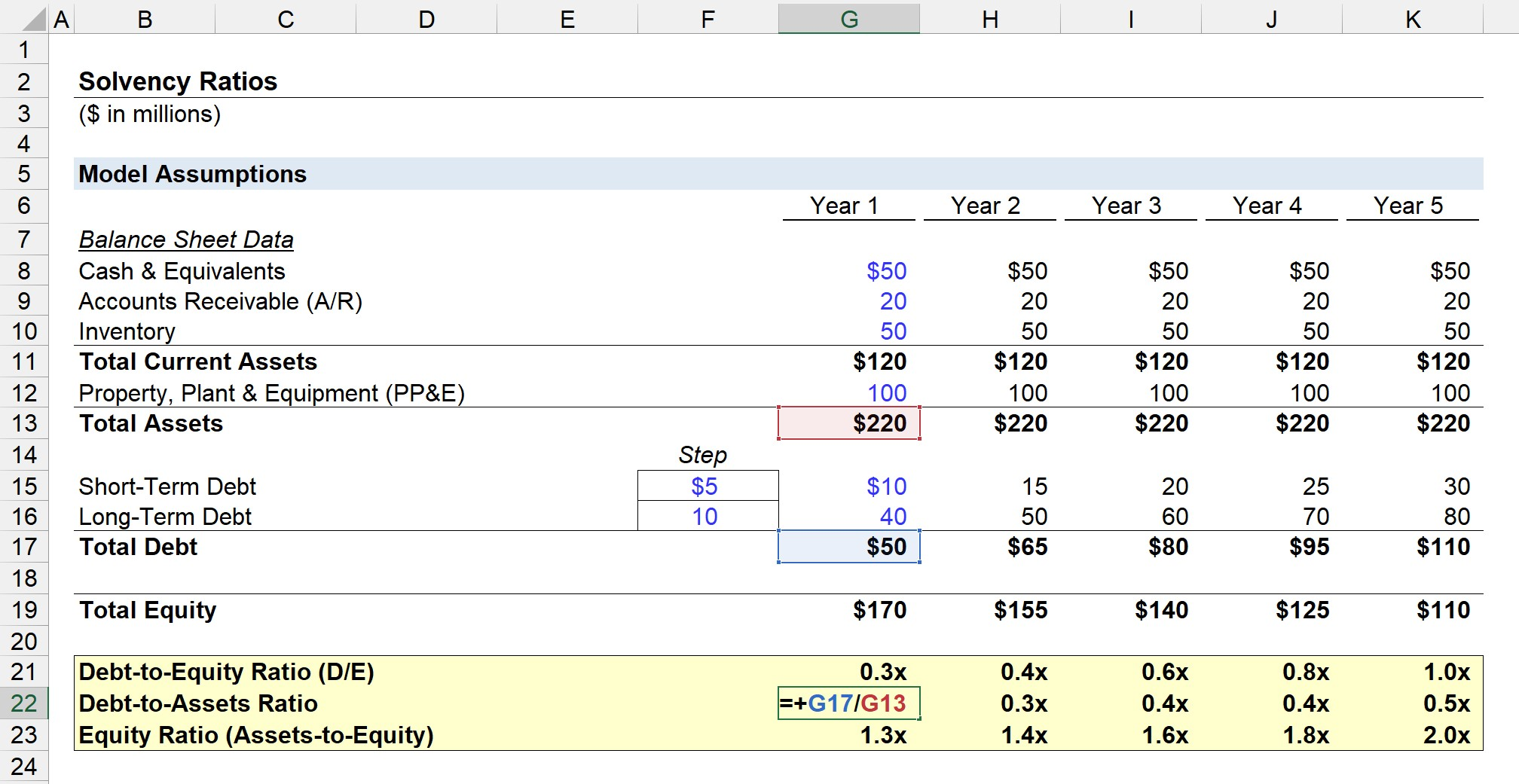## 4. Equity Ratio Calculation Analysis

As for our final solvency metric, the equity ratio is calculated by dividing total assets by the total equity balance.

In Year 1, we arrive at an equity ratio of 1.3x.

• Equity Ratio = \$220m / \$170m = 1.3x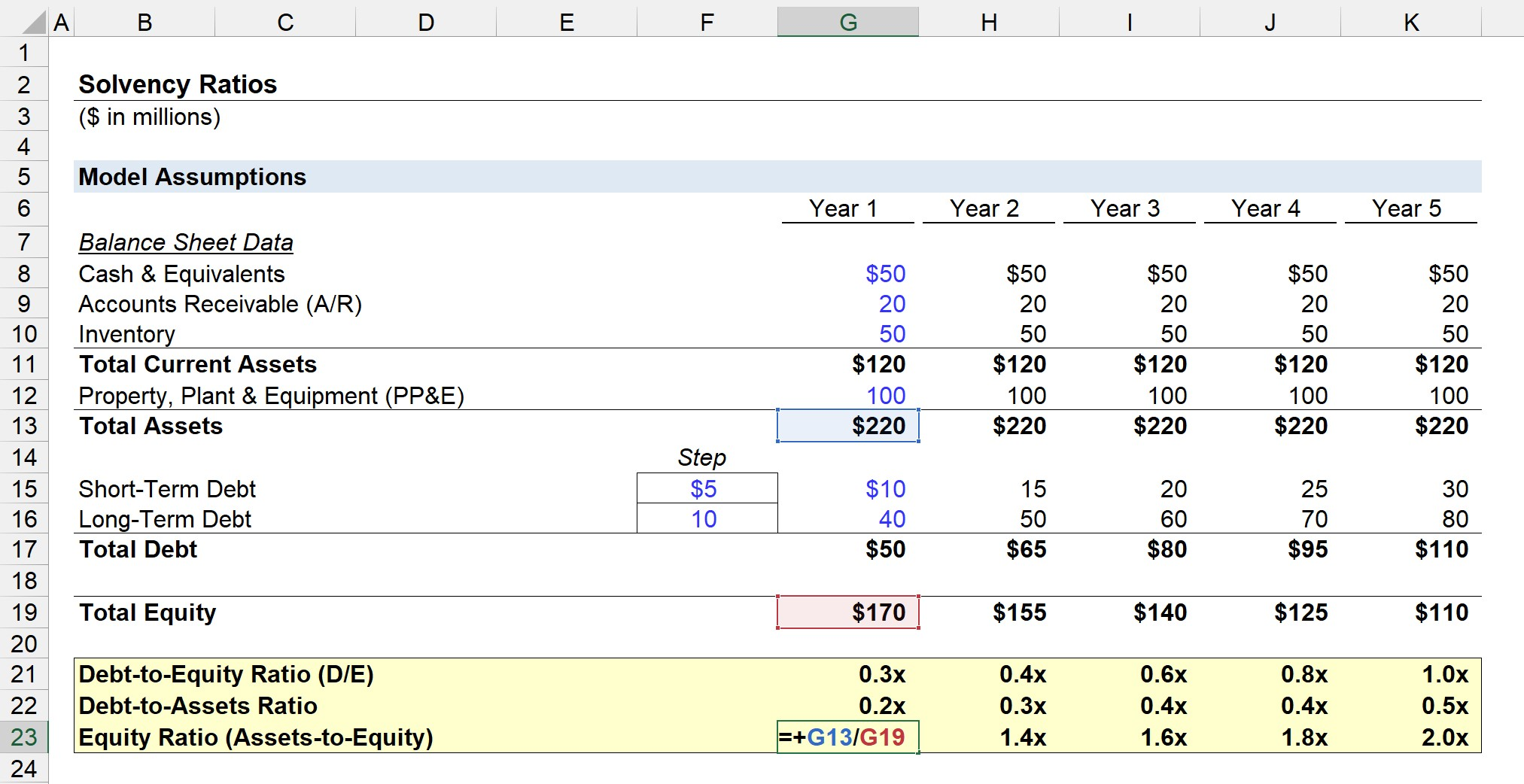## 5. Solvency Ratio Calculation Example

From Year 1 to Year 5, the solvency ratios undergo the following changes.

• D/E Ratio: 0.3x → 1.0x
• Debt-to-Assets Ratio: 0.2x → 0.5x
• Equity Ratio: 1.3x → 2.0x

By the end of the projection, the debt balance is equal to the total equity (i.e. 1.0x), showing that the company’s capitalization is evenly split between creditors and equity holders on a book value basis.

The debt-to-assets ratio increases to approximately 0.5x, which means the company must sell off half of its assets to pay off all of its outstanding financial obligations.

And finally, the equity ratio increases to 2.0x, as the company is incurring more debt each year to finance the purchase of its assets and operations.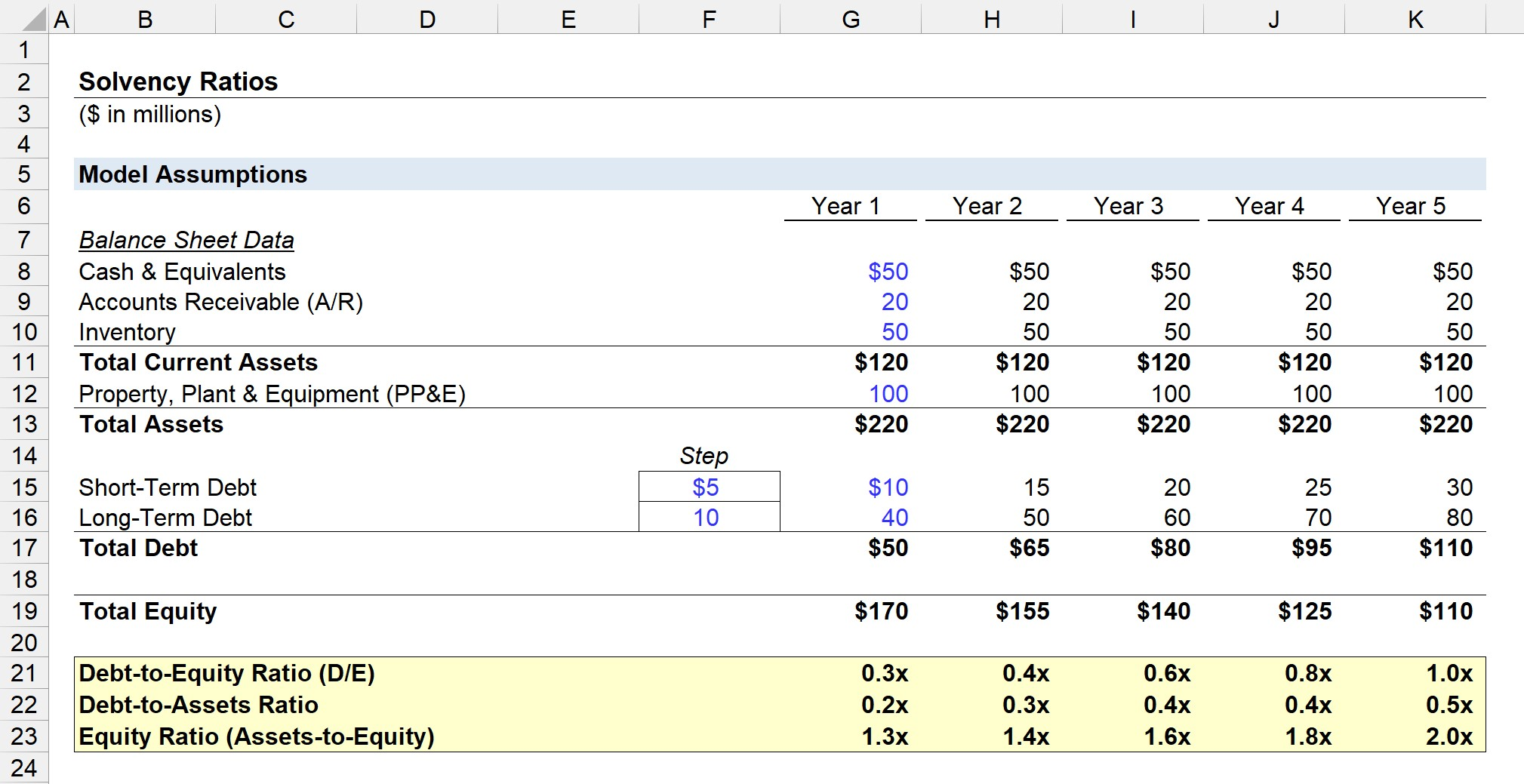Step-by-Step Online Course

### Everything You Need To Master Financial Modeling

Enroll in The Premium Package: Learn Financial Statement Modeling, DCF, M&A, LBO and Comps. The same training program used at top investment banks.